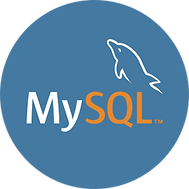top of page# CONVERT

## База данных: MySQL## Topic

Syntax:
CONVERT(expr USING transcoding_name), CONVERT(expr,type)

The CONVERT() function takes an expression of any type and produces a
result value of the specified type.

CONVERT(... USING ...) is standard SQL syntax. The non-USING form of
CONVERT() is ODBC syntax.

CONVERT(expr USING transcoding_name) converts data between different
character sets. In MySQL, transcoding names are the same as the
corresponding character set names. For example, this statement converts
the string 'abc' in the default character set to the corresponding
string in the utf8mb4 character set:

SELECT CONVERT('abc' USING utf8mb4);

CONVERT(expr, type) syntax (without USING) takes an expression and a
type value specifying the result type. This operation may also be
expressed as CAST(expr AS type), which is equivalent. These type values
are permitted:

o BINARY[(N)]

Produces a string with the BINARY data type. For a description of how
this affects comparisons, see
https://dev.mysql.com/doc/refman/8.0/en/binary-varbinary.html. If the
optional length N is given, BINARY(N) causes the cast to use no more
than N bytes of the argument. Values shorter than N bytes are padded
with 0x00 bytes to a length of N.

o CHAR[(N)] [charset_info]

Produces a string with the CHAR data type. If the optional length N
is given, CHAR(N) causes the cast to use no more than N characters of
the argument. No padding occurs for values shorter than N characters.

With no charset_info clause, CHAR produces a string with the default
character set. To specify the character set explicitly, these
charset_info values are permitted:

o CHARACTER SET charset_name: Produces a string with the given
character set.

o ASCII: Shorthand for CHARACTER SET latin1.

o UNICODE: Shorthand for CHARACTER SET ucs2.

In all cases, the string has the character set default collation.

o DATE

Produces a DATE value.

o DATETIME

Produces a DATETIME value.

o DECIMAL[(M[,D])]

Produces a DECIMAL value. If the optional M and D values are given,
they specify the maximum number of digits (the precision) and the
number of digits following the decimal point (the scale).

o DOUBLE

Produces a DOUBLE result. Added in MySQL 8.0.17.

o FLOAT[(p)]

If the precision p is not specified, produces a result of type FLOAT.
If p is provided and 0 <= < p <= 24, the result is of type FLOAT. If
25 <= p <= 53, the result is of type DOUBLE. If p < 0 or p > 53, an
error is returned. Added in MySQL 8.0.17.

o JSON

Produces a JSON value. For details on the rules for conversion of
values between JSON and other types, see
https://dev.mysql.com/doc/refman/8.0/en/json.html#json-comparison.

o NCHAR[(N)]

Like CHAR, but produces a string with the national character set. See
https://dev.mysql.com/doc/refman/8.0/en/charset-national.html.

Unlike CHAR, NCHAR does not permit trailing character set information
to be specified.

o REAL

Produces a result of type REAL. This is actually FLOAT if
REAL_AS_FLOAT SQL mode is enabled; otherwise the result is of type
DOUBLE.

o SIGNED [INTEGER]

Produces a signed integer value.

o TIME

Produces a TIME value.

o UNSIGNED [INTEGER]

Produces an unsigned integer value.

o YEAR

Produces a YEAR value. Added in MySQL 8.0.22. The rules governing
conversion to YEAR are listed here:

o For a four-digit number in the range 1901-2155 inclusive, or for a
string which can be interpreted as a four-digit number in this
range, return the corresponding YEAR value.

o For a number consisting of one or two digits, or for a string which
can be interpeted as such a number, return a YEAR value as follows:

o If the number is in the range 1-69 inclusive, add 2000 and return
the sum.

o If the number is in the range 70-99 inclusive, add 1900 and
return the sum.

o For a string which evaluates to 0, return 2000.

o For the number 0, return 0.

o For a DATE, DATETIME, or TIMESTAMP value, return the YEAR portion
of the value. For a TIME value, return the current year.

If you do not specify the type of a TIME argument, you may get a
different result from what you expect, as shown here:

mysql> SELECT CONVERT("11:35:00", YEAR), CONVERT(TIME "11:35:00", YEAR);
+---------------------------+--------------------------------+
| CONVERT("11:35:00", YEAR) | CONVERT(TIME "11:35:00", YEAR) |
+---------------------------+--------------------------------+
| 2011 | 2020 |
+---------------------------+--------------------------------+

o If the argument is of type DECIMAL, DOUBLE, DECIMAL, or REAL, round
the value to the nearest integer, then attempt to cast the value to
YEAR using the rules for integer values, as shown here:

mysql> SELECT CONVERT(1944.35, YEAR), CONVERT(1944.50, YEAR);
+------------------------+------------------------+
| CONVERT(1944.35, YEAR) | CONVERT(1944.50, YEAR) |
+------------------------+------------------------+
| 1944 | 1945 |
+------------------------+------------------------+
1 row in set (0.00 sec)

mysql> SELECT CONVERT(66.35, YEAR), CONVERT(66.50, YEAR);
+----------------------+----------------------+
| CONVERT(66.35, YEAR) | CONVERT(66.50, YEAR) |
+----------------------+----------------------+
| 2066 | 2067 |
+----------------------+----------------------+
1 row in set (0.00 sec)

o An argument of type GEOMETRY cannot be converted to YEAR.

o For a value that cannot be successfully converted to YEAR, return
NULL.

A string value containing non-numeric characters which must be
truncated prior to conversion raises a warning, as shown here:

mysql> SELECT CONVERT("1979aaa", YEAR);
+--------------------------+
| CONVERT("1979aaa", YEAR) |
+--------------------------+
| 1979 |
+--------------------------+
1 row in set, 1 warning (0.00 sec)

mysql> SHOW WARNINGS;
+---------+------+-------------------------------------------+
| Level | Code | Message |
+---------+------+-------------------------------------------+
| Warning | 1292 | Truncated incorrect YEAR value: '1979aaa' |
+---------+------+-------------------------------------------+

URL: https://dev.mysql.com/doc/refman/8.0/en/cast-functions.html

bottom of page